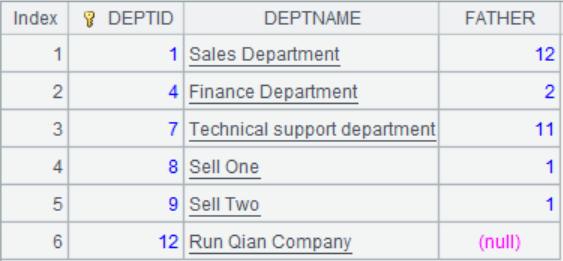# ym_importance ()

ym_importance(pd)

 pd pd模型对象

 A 1 =ym_env() 启动python服务 2 =file("train.csv") 3 =ym_model(A1,A2) 加载建模数据文件 4 =ym_target(A3, "Survived") 设置目标变量 5 =ym_build_model(A3) 执行建模操作，返回模型对象 6 =ym_importance(A5) 获取模型变量的重要度列表7 >ym_close(A1)

 A 1 =ym_env() 启动python服务 2 =ym_load_pcf("ttnkh.pcf") 根据模型文件生成模型对象，ttnkh.pcf为已存在的模型文件 3 =ym_importance(A2) 获取模型变量的重要度列表 4 >ym_close(A1) 释放资源